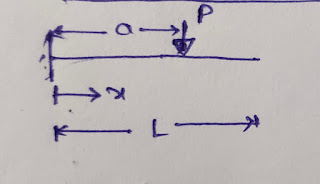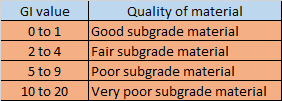## Translate

### SUPERPOSITION METHOD/ METHOD OF TABLES OF FINDING DEFLECTION

• Table method of finding deflection of beam is one of the best method of finding deflection of beam.
• This method of finding deflection is very much beneficial in case of finding slope and deflection as per superposition method.
• As per superposition method , the effect of each and every load is considered separately and finally the effect of each loading is summed up.
• This method is even very much helpful in case of analysis of indeterminate structure with the force method of analysis. By choosing the redundant , the effect of various loading is calculated at the desired position with the help of the table.
• The table consists of slope and deflection equation of some standard type of beam under various standard loadings.

##• For 0 ≤ x  a
Ө = (P/2EI) (X² - 2aX)

y = (P/6EI) (X³ - 3aX²)

• For a ≤ x ≤ L

Ө = - Pa²/2EI

y = (Pa² / 6EI) (a - 3x)

## Ө = - Mx/EI

y = - Mx²/ 2EI

• For a ≤ x ≤ L
• ## Ө = -Ma/EI

y = (Ma/2EI) (a - 2x)

## Ө =(w/6EI)(3ax²-3a²x-x³)

y = (w/24EI)(4ax³-6a²x²-x⁴)

•  For a ≤ x ≤ L

## Ө = - wa³/6EI

y = (wa³/24EI) (a - 4x)

## Ө = (w/24EIa)(x⁴-4ax³+6a²x²-4a³x)

= (w/120EIa)(x⁵-5ax⁴+10a²x³-10a³x²)

•  For a ≤ x ≤ L

## Ө = (- wa³/24EI)

y = (wa³ /120EI)( -5x+a)

## Ө = (Pb/6EIL)(3x²+b²-L²)

= (Pb/6EIL)(x³+b²x-L²x)

•  For a ≤ x ≤ L

## Ө = (Pa/6EIL)(L²-a²-3(L-x)²)

y = (Pa(L-x)/6EIL)(x²+a²-2Lx)

## Ө = (M/6EIL)(-3x²+6aL-3a²-2L²)

y = (M/6EIL)(-x³+6aLx-3a²x-2L²x)

## Ө = (-w/24EIL)[4Lx³-6a(2L-a)x²+a²(2L-a)²]

y = (-w/24EIL)[Lx⁴-2a(2L-a)x³+a²(2L-a)²x]

## Ө = (-wa²/24EIL)(6x²-12Lx+a²+4L²)

y = (-wa²/24EIL)(L-x)(-2x²+4Lx-a²)

## Ө = (-w/360EIL)(15x⁴-30L²x²+7L⁴)

y = (-w/360EIL)(3x⁵-10L²x³+7L⁴x)